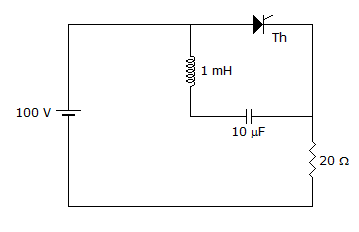# Electronics and Communication Engineering - Power Electronics

### Exercise :: Power Electronics - Section 5

6.

A bridge type single phase cycloconverter changes the frequency f to f/3. Then one half wave of output contains

 A. 3 full waves of input B. 3 half waves of input C. 6 full waves of input D. 6 half waves of input

Explanation:

No answer description available for this question. Let us discuss.

7.

A half bridge inverter

 A. needs a 3 wire dc supply B. may use a two wire or 3 wire dc supply C. has higher output voltage if fed from 3 wire dc supply D. has higher efficiency if fed from 3 wire dc supply

Explanation:

No answer description available for this question. Let us discuss.

8.

A 3 phase series inverter has

 A. 12 thyristors B. 6 thyristors C. 3 thyristors D. 3 or 6 thyristors

Explanation:

No answer description available for this question. Let us discuss.

9.

In figure, thyristor Th is in off state. When thyristor is turned on, the peak thyristor current can beA. 5 A B. 10 A C. 15 A D. 20 A

Explanation:

No answer description available for this question. Let us discuss.

10.

HVDC systems are preferable for only long distances.

 A. True B. False